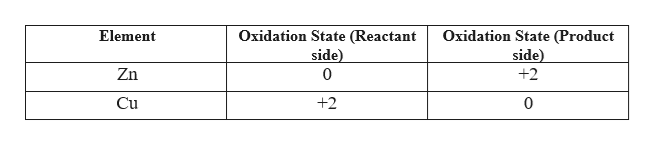# a. Balance the following equations. b. Identify oxidizing and reducing agents. c. Determine the oxidation numbers of the elements involved in the redox reaction.Zn(s) + CuSO4(aq) ---->  I2(s) + S2O32-(aq) -----> I-(aq) + S4O62-(aq)

Question
5 views

a. Balance the following equations. b. Identify oxidizing and reducing agents. c. Determine the oxidation numbers of the elements involved in the redox reaction.

Zn(s) + CuSO4(aq) ---->

I2(s) + S2O32-(aq) -----> I-(aq) + S4O62-(aq)

check_circle

Step 1

The balanced chemical equation is given as

Step 2

Oxidising agents are those which gets reduced i.e. their oxidation state decreases as the reaction progresses where as reducing agents are those which gets oxidised i.e. their oxidation state increases in the reaction.

Here, the oxidation states of the elements are given as,help_outlineImage TranscriptioncloseOxidation State (Reactant side) 0 Oxidation State (Product side) Element Zn +2 Cu +2 0 fullscreen
Step 3

Hence, Zn is reducing agent and ...

### Want to see the full answer?

See Solution

#### Want to see this answer and more?

Solutions are written by subject experts who are available 24/7. Questions are typically answered within 1 hour.*

See Solution
*Response times may vary by subject and question.
Tagged in
ScienceChemistry

### Redox Reactions